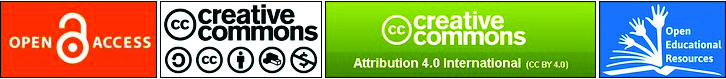### COMPARING OF MATHEMATICS TEACHERS CANDIDATES’ MATHEMATICAL MODELLING SKILLS: TURKEY AND ENGLAND SAMPLES

Alattin Ural

#### Abstract

This study aims to compare mathematical modelling abilities of prospective mathematics teachers. 38 Turkish prospective mathematics teachers studying at the Department of Primary Mathematics Education and 26 English prospective mathematics teachers studying at Initial Mathematics Teacher Education Program of the Graduate School of Education attended the study. Two mathematical modelling problems were given to the students. The stages of mathematical modelling given by Berry and Houston (1995) were used to examine the participants’ mathematical modelling abilities. What the Turkish and English students did in each of these stages was presented separately for both problems categorically and with the percentage tables and to what extent the students were successful in these stages was shown. The achievement scores of Turkish and English students were compared with independent groups t-test. The results showed that almost all of the participants were quite inadequate especially at the point of setting the mathematical model. This result indicates that mathematics teacher candidates had difficulty in transferring algebraic concepts such as function, equation, inequality to real-life problems. On the other hand, it can be said that English participants' abilities were better than that of Turkish participants.

Article visualizations:#### Keywords

mathematical modelling; modelling competency; prospective mathematics teacher

PDF

#### References

Berry .J, & Houston, K. (1995). Mathematical modelling. Bristol, J. W: Arrowsmith Ltd.

Blum, W., Galbraith, P., Henn, H., & Niss, M. (Eds.) (2007). Modelling and applications in mathematics education: New ICMI studies series,10. New York: Springer.

Blum W., Ferri, B. R. (2009). Mathematical modelling: can it be taught and learnt?, Journal of Mathematica Modelling and Application, 1(1), 45-58.

Busse, A. (2001). Zur Rolle des Sachkontextes bei realitätsbezogenen mathematikaufgaben. In G. Kaiser (Ed.), Beiträge zum Mathematikunterricht, (pp. 141-144). Hildesheim: Franzbecker.

Christiansen, I. (2001). The effect of task organization on classroom modelling activities. In J. Matos, W. Blum, K. Houston, & S. Carreira (Eds.), Modelling and Mathematics Education, ICTMA 9: Applications in Science and Technology, (pp. 311-320). Chichester: Horwood Publishing.

Crouch, R., & Haines, C. (2004). Mathematical modelling: transitions between the real world and the mathematical model, International Journal of Mathematical Education in Science and Technology, 35(2), 197-206.

Erdoğan, A. (2010). Primary teacher education students’ ability to use functions as modelling tools. Procedia Social and Behavioral Sciences, 2, 4518-4522.

Galbraith, P., & Stillman, G. (2001). Assumptions and context: Pursuing their role in modelling activity. In J. F. Matos, S. K. Houston, W. Blum & S. P. Carreira (Eds.), Mathematical Modelling and Mathematics Education: Applications in Science and Technology. Chichester: Horwood Publishing.

Haines, C., Crouch, R., & Davies, J. (2001). Understanding students’ modelling skills. In J. Matos, W. Blum, K. Houston & S. Carreira (Eds.), Modelling and Mathematics Education, ICTMA 9: Applications in Science and Technology, (pp. 366-380). Chichester: Horwood Publishing.

Hodgson, T. (1997). On the use of open-ended, real-world problems. In K. Houston, W. Blum, I. Huntley, N.T. Neill (Eds.), Teaching and Learning Mathematical Modelling, (pp. 211-218). Chichester: Albion publishing limited.

Houston, K. (Ed) (2005). Applications and modelling in the teaching and learning of mathematics, ICME-10, Teaching Mathematics and its Applications, 24 (2/3).

Kaiser, G. (2005). Mathematical modelling in school: examples and experiences. In Henn, H. W. & Kaiser, G. (Eds.), Mathematikunterricht im Spannungsfeld von Evolution und Evaluation. Festband für Werner Blum. Hildesheim: Franzbecker.

Kaiser, G. (2007). Modelling and modelling competencies in school. In C. Haines, P. Galbraith, W. Blum & S. Khan (Eds.), Mathematical modelling, ICTMA 12: Education, Engineering and Economics: Proceedings from the Twelfth International Conference on the Teaching of Mathematical Modelling and Applications, (pp. 110-119). Chichester: Horwood.

Kaiser, G., Blomhoj, M., & Sriraman, B. (2006). Towards a didactical theory for mathematical modelling, ZDM, 38(2), 82-85.

Klymchuk, S. S. & Zverkova T. S. (2001). Role of mathematical modelling and applications in university service courses: An across countries study. In J. F. Matos, W. Blum, S. K. Houston & S. P. Carreira (Eds.), Modelling, Applications and Mathematics Education-Trends and Issues, (pp. 227-235). Chichester UK: Ellis Horwood.

Lingefjärd, T. (2006). Faces of mathematical modelling, Zentralblatt für Didaktik der Mathematik, 38(2), 96-112.

Niss, M. (2001). University mathematics based on problem-oriented student projects: 25 years of experiences with the Roskilde model. In D. Holton (Ed.), The Teaching and Learning of Mathematics at University Level: An ICMI Study, (pp. 405-422). Dordrecht: Kluwer Academic Publishers.

Niss, M. (2003). Mathematical competencies and the learning of mathematics: The Danish KOM Project. In A. Gagatsis, & S. Papastavridis (Ed), 3rd Mediterranean Conference on Mathematical Education, (pp. 115–124). Athens: The Hellenic Mathematical Society.

OECD (2007). PISA 2006-Science competencies for tomorrow's world, Vol. 1&2. Paris: OECD.

Tanner, H. & Jones, S. (1995). Developing metacognitive skills in mathematical modelling-a socio-constructivist interpretation. In C. Sloyer, W. Blum & I. Huntley (Eds.), Advances and Perspectives in the Teaching of Mathematical Modelling and Applications, (pp. 61-70). Yorklyn: Water Street Mathematics.

Türker, B., Sağlam, Y. & Umay, A. (2010). Preservice teachers’performances at mathematical modelling process and views on mathematical modelling, Procedia Social and Behavioral Sciences 2, 4622-4628.

Verschaffel, L., De Corte, E. & Borghart, I. (1997). Pre-service teachers’conceptions and beliefs about the role of real-world knowledge in mathematical modelling of school word problems, Learning and Instruction, 7(4), 339-359.

Zeytun, A., Çetinkaya, B., Yıldırım, U. & Erbaş, A. K. (2009, July). Pre-service physics teachers’ difficulties in constructing mathematical models of simple harmonic motion. Paper presented at the 14th International Conference on the Teaching of Mathematical Modelling and Applications (ICTMA-14), Hamburg, Germany.

DOI: http://dx.doi.org/10.46827/ejes.v0i0.2754

### Refbacks

• There are currently no refbacks.